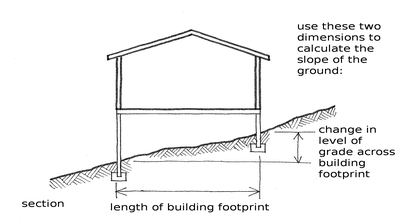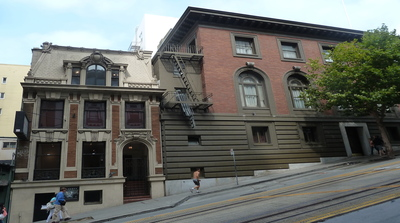The slope of the ground is input where the building is located on a sloping site, where the height of the ground floor above grade varies by more than 1 m. The slope of the building is measured across the length of the building footprint, in the direction where the greatest difference in level is observed. The slope is measured as an angle from horizontal (expressed in degrees), where a flat site would be zero (0) degrees.

For flat sites, where the variation of height of ground floor level above grade is less than 1m, the default value is zero (0) degrees.

The slope of ground can be specified as follows:

HD:a                       (where a denotes slope in degrees)

Example: HD:10 (the slope is 10 degrees)The slope of the ground is calculated from the length of the building footprint (in the direction where the greatest difference in level is observed), and the change in level of grade across this length.The slope of ground for these buildings in San Francisco, California is around 12 degrees, that is, HD:12